# Smooth Connection on a Free Thin Square PlateThis test lets you study the smooth connection in the case of a modal analysis. You will use 2D meshes.Reference:

Guide de validation des prologiciels de calcul des structures, AFNOR Technique, pp216-217, SFM.

##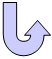Specifications

### Geometry Specifications

 Length: L = 1000 mm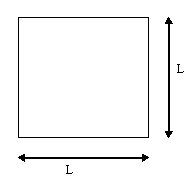Thickness: th = 10 mm

### Analysis Specifications

 Young Modulus (material): E = 210 GPa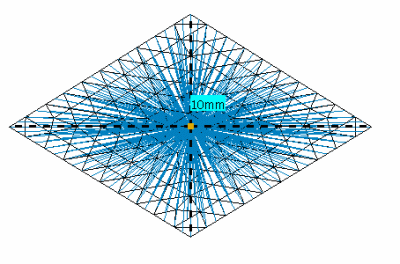Mesh formed of parabolic triangle elements (TR6), with a smooth connection. Poisson's Ratio (material): ν = 0.3 Density: ρ = 7800 kg/m3 Mesh Specifications: 10 x 10 elements Restraints: smooth connection case: a clamp on the point where the smooth connection is applied. no smooth connection case: no restraint

##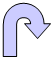Results

This test presents the modal frequencies of a plate with a smooth connection in its center, and compares these results with the semi-analytical solution of M.V. Barton (results A). The modal frequencies of the smoothly-connected plate are also compared with the frequencies of a plate without connection (results B).

### Reference Solutions

• Results A: Semi-analytical solution for frequencies of M.V. Barton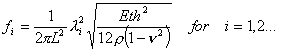with :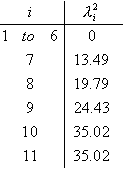• Results B: Computed values for a plate without connection: Mode* Semi-analytical solution of M.V. Barton Computed values Linear triangle (TR3) Parabolic triangle (TR6) Linear quadrangle (QD4) Parabolic quadrangle (QD8)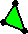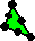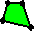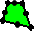7 33.7119 33.5005 33.3576 33.6410 33.5163 8 49.4558 49.2273 48.9560 49.3634 48.9541 9 61.0513 61.1598 60.6258 61.4091 60.6241 10 87.5160 87.3410 86.2420 87.5727 86.5851 11 87.5160 87.3510 86.2586 87.5727 86.5851

* On a plate without connection, the six first modes of a free modal analysis are rigid. With a smooth connection, these modes do not exist, because of the clamp of the connection. We compare, for the three solutions, the results of the five first free modes.

### Computed Results of the Plate with a Smooth Connection

•  Comparison with Results A Mode* Computed values Linear triangle (TR3) Parabolic triangle (TR6) Linear quadrangle (QD4) Parabolic quadrangle (QD8)Computed solution Comparison with results A  [% of error] Computed solution Comparison with results A  [% of error] Computed solution Comparison with results A  [% of error] Computed solution Comparison with results A  [% of error] 1 33.5005 0.63 33.3575 1.05 33.641 0.21 33.5163 0.58 2 49.2271 0.46 48.9557 1.01 49.3634 0.19 48.9541 1.01 3 59.8521 1.96 60.3319 1.18 59.5249 2.50 59.6750 2.25 4 86.6717 0.96 86.1295 1.58 86.6419 1.00 86.1747 1.53 5 86.7038 0.93 86.1464 1.56 86.6419 1.00 86.1747 1.53

• Comparison with Results B Mode* Computed values Linear triangle (TR3) Parabolic triangle (TR6) Linear quadrangle (QD4) Parabolic quadrangle (QD8)Computed solution Comparison with results B  [% of error] Computed solution Comparison with results B  [% of error] Computed solution Comparison with results B  [% of error] Computed solution Comparison with results B  [% of error] 1 33.5005 0.00 33.3575 0.00 33.641 0.00 33.5163 0.00 2 49.2271 0.00 48.9557 0.00 49.3634 0.00 48.9541 0.00 3 59.8521 2.14 60.3319 0.48 59.5249 3.07 59.6750 1.57 4 86.6717 0.77 86.1295 0.13 86.6419 1.06 86.1747 0.47 5 86.7038 0.74 86.1464 0.13 86.6419 1.06 86.1747 0.47

* On a plate without connection, the six first modes of a free modal analysis are rigid. With a smooth connection, these modes do not exist, because of the clamp of the connection. We compare, for the three solutions, the results of the five first free modes.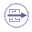To Perform the Test:

The assembly_connection_tr6_smooth.CATAnalysis document presents a complete analysis of this case, computed with a mesh formed of parabolic triangle elements (TR6), with a smooth connection.

The assembly_connection_tr6_no_smooth.CATAnalysis document presents a complete analysis of this case, computed with a mesh formed of parabolic triangle elements (TR6) without a smooth connection.

To compute these two cases with other meshes, proceed as follow:

1. Open one of the CATAnalysis documents.

2. Enter the Advanced Meshing Tools workbench.

3. In the specification tree, double-click on the mesh:
The Global Parameters dialog box appears.

4. Change the mesh specifications as indicated below.

5. Compute the case in the Generative Structural Analysis workbench.